# GSEB Solutions Class 9 Maths Chapter 14 Statistics Ex 14.4

Gujarat Board GSEB Solutions Class 9 Maths Chapter 14 Statistics Ex 14.4 Textbook Questions and Answers.

## Gujarat Board Textbook Solutions Class 9 Maths Chapter 14 Statistics Ex 14.4

Question 1.
The following number of goals were scored by a team in a series of 10 matches:
2, 3, 4, 5, 0, 1, 3, 3,4,3.
Find the mean, median, and mode of these scores.
Solution:
1. Mean: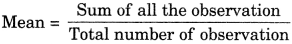$$\frac{2+3+4+5+0+1+3+3+4+3}{10}$$
$$\frac{28}{10}$$ = 2.8

2. Median:
Arranging the given data in ascending order, we have
0, 1, 2,3,3,3,4,4, 5.
Number of observations (n) = 10, which is even.
∴ Median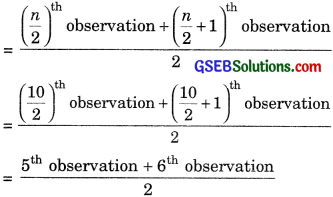$$\frac{3+3}{2}=\frac{6}{2}$$ = 3

3. Mode:
Arranging the given data in ascending order, we have
0,1,2,3,3,3,3,4,4, 5.
Here, 3 occurs most frequently (4 times)
∴ Mode = 3.Question 2.
In a mathematics test given to 15 students, the following marks (out of 100) are recorded:
41, 39, 48, 52, 46, 62, 54, 40, 96, 52, 98, 40, 42, 52, 60.
Find the mean, median, and mode of this data.
Solution:
1. Mean: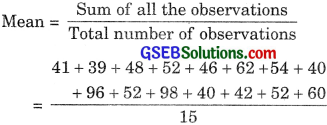$$\frac{822}{15}$$ = 54.8

2. Median:
Arranging the given data in ascendin order, we have
39, 40, 40, 41, 42, 46, 48, 52, 52, 52, 54, 60, 62, 96, 98.
Number of observations (n) = 15, which is odd.

∴ Median = $$\left(\frac{n+1}{2}\right)^{t h}$$ observation
= $$\left(\frac{15+1}{2}\right)^{th}$$
= 8th observation = 52.

3. Mode:
Arranging the given data in descending or der, we have
98, 96, 62, 60, 54, 52, 52, 52, 48, 46, 42, 41, 40, 40, 39.
Here, 52 occurs most frequently (3 times)
∴ Mode = 52.Question 3.
The following observations have been arranged in ascending order. If the median of the data is 63, find the value of x.
29, 32, 48, 50, x, x + 2, 72, 78, 84, 95.
Solution:
Number of observations (n) = 10, which is even.
∴ Median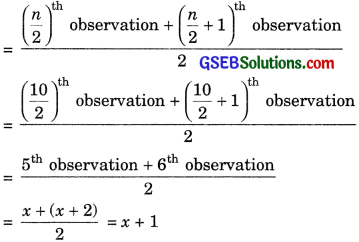According to the questions,
x + 1 = 63 ⇒ x = 63 – 1 ⇒ x = 62
Hence, the value of x is 62.

Question 4
Find the mode of 14, 25, 14, 28, 18, 17, 18, 14, 23, 22, 14, 18.
Solution:
Arranging the given data in ascending order, we have
14, 14, 14, 14, 17, 18, 18, 18, 22, 23, 25, 28
Here 14 occurs most frequently (4 times)
∴ Mode = 14.Question 5.
Find the mean salary of 60 workers of a factory from the following table: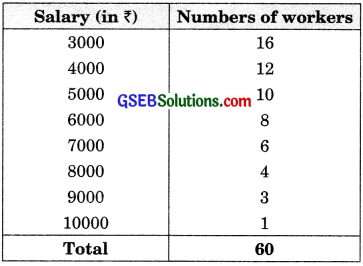Solution: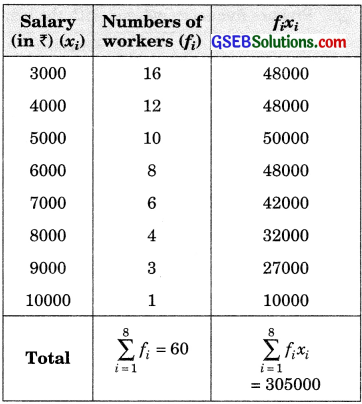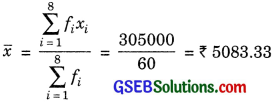Hence, the mean salary is ₹ 5083.33.

Question 6.
Give one example of a situation in which:

• the mean is an appropriate measure of central tendency.
• the mean is hot an appropriate measure of central tendency but the median is an appropriate measure of central tendency.

Solution:

• mean marks in a test in mathematics.
• average beauty.